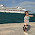### leetcode Question 124: Word Search

Word Search

Given a 2D board and a word, find if the word exists in the grid.
The word can be constructed from letters of sequentially adjacent cell, where "adjacent" cells are those horizontally or vertically neighboring. The same letter cell may not be used more than once.
For example,
Given board =
[
["ABCE"],
["SFCS"],
]

word = "ABCCED", -> returns true,
word = "SEE", -> returns true,
word = "ABCB", -> returns false.

Analysis:
The idea of this question is as follows:
(1) Find the 1st element of the word in the board.
(2) For each position found where the 1st element lies, recursively do:
(i) Search the around cell to see if the next element exists. (4 directions: (i-1,j),(i+1,j),(i,j-1),(i,j+1) )
(ii) If the word ends, return true.
(3) Return false if no matching found.
Note: A mask matrix is needed to store the positions where have already been visited. Details can be found in code.

Code:

class Solution {
public:

bool search(vector<vector<char> > &board, int i,int j,string str,vector<vector<bool> > &mask){
if (str.size()==0){return true;}
else{
return true;
}
}
return true;
}
}
return true;
}
}
return true;
}
}
}
return false;
}

bool exist(vector<vector<char> > &board, string word) {
// Start typing your C/C++ solution below
// DO NOT write int main() function
if (word.size()==0) {return false;}

for (int i=0;i<board.size();i++){
for (int j=0;j<board.size();j++){
if (word==board[i][j]){
if (word.size()==1) {return true;}
else{
return true;
}
}
}
}
}
return false;
}
};


1.1.2.